HistoryMathematiciansAll formulasNum. approx.SoftwaresMisc. math.DigitsPoetryPapers/videosDelirium !Pi-DayImagesMusicLinksBibliography Boris Gourévitch The world of Pi - V2.57 modif. 13/04/2013HomeVersion historyGuestbookWho I amSome pictures (fr)AcknowledgementsLast modificationsContact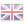Isaac Newton

## Newton’s method

### 1 The roots of the problem

Let’s imagine that you’re asked for the first digits of. Silence for a few seconds... mmmh... well, it’s above 1, less than 2 because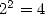... It’s less than 1.5 since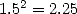etc... Nothing very simple... So how could the mathematicians of the 17th century numerically compute the roots of a 4th degree polynomial ?

Always prolific, Newton proposed around 1669 (!) an iterative algorithm converging quadratically towards roots of a number. Quadratically means that the number of correct digits of the numerical estimate doubles at each step. Thenceforth, algorithm complexity of the division and roots extraction becomes equal to that of multiplication. A godsend for modern computations, where bruising roots extractions are experienced in the algorithms of Salamin  or those from the Borwein  brothers. The Newton’s algorithm a several qualities, which are exposed in the following paragraphs.

### 2 Wording of Newton’s method

Originally, i.e. more than three centuries ago, Newton’s method was used to numerically estimate a rootin the equation. Actually, this algorithm allows to show the complexity equivalence of multiplication, division and roots extraction.

In 1669, Newton proposed an iterative algorithm to find roots of polynomial (Newton’s iteration). Then, Raphson reworded it in a more modern way in 1690. Simpson, Mourraille, Cauchy and Kantorovich completed it and made it more general, which finally gives the following wording:

Theorem 2.1 Let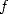be analytic in a complex neighboring of. Let’s suppose thatand. Then, the following algorithm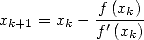(1)

uniformly and quadratically converges towardswhen the initial valueis chosen to be in a neighboring of.

This algorithm has an amazing property of errors autocompensation : ifis modified such that only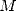correct digits are left compared tothenwill have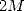correct digits though, given that enough digits are used at this step. In other words, the quadratic convergence is preserved.

One straightforward consequence is that the computation oftodigits only require to initialize the algorithm with a couple of correct digits, then double the number of digits taken into account at each step until at leastdigits are reached. This dramatically reduces the complexity of the computation and allows to show that multiplication, division and roots extraction are equivalent.

### 3 Applications

#### 3.1 Multiplicative inverse of a number

Let’s choose a number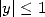. We want to compute its multiplicative inverse with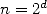correct digits. Using the function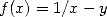, the Newton’s algorithm becomes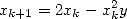(2)

which is only using multiplications and additions. The equation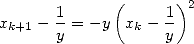(3)

illustrates the quadratic convergence of the algorithm. Suppose now that we initialize the algorithm with one correct digit. We then have(4)

From the errors autocompensation property of the Newton’s method, we are allowed to only use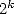digits at theth step. And from 2, we only needmultiplications (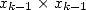et) andadditions (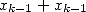et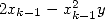) to obtain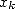. Thanks to the quadratic convergence, onlyitérations of 2 are needed to obtaincorrect digits of. If we defineas being the complexity of a multiplication method applied todigits, given that the complexity of an addition will be, we then obtain a final complexity of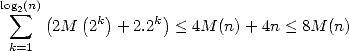(5)

for the multiplicative inverse computation. Indded, we can reasonably suppose that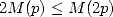, which means that the complexity ofmultiplications of numbers with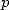digits is slightly less complex than the multiplication of two numbers with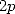digits. From this ensues that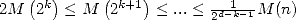in 5. Thus, the multiplicative inverse is not far more complex than multiplication (it’s a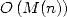). Moreover, since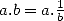, division also is a.

#### 3.2 Square root extraction

Things also are going well. We can use the functionwhich leads to the estimation of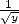then we use the fact that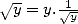. The associated iteration is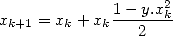(6)

which practically leads to a final complexity of around.

A natural alternative uses directly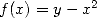, but this leads to an iteration showing a division: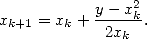(7)

However, the prolific Schönhage, full of ideas (see also FFT), proposed to replacewith another iteration in 1971. He then obtained the following coupled iteration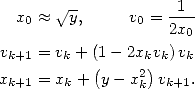estimates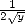. this algorithm is called “Newton’s coupled iteration” (, p257) and practically gives a complexity of around.

You can find several refinements of Newton’s method in . The complexity equivalence of multiplication, division and roots extraction is exposed in .

### References

   J. Arndt, C. Haenel, ”Unleashed”, Springer-Verlag, Berlin Heidelberg New York, 2001.

   R. Brent, “Fast multiple-precision evaluation of elementary fonctions”, Journal of the Association of Computing Machinery, 1976, pp.242-251.

   S. A. Cook, S. O. Aanderaa, “On the minimum computation of functions”, Trans. AMS, 1969, vol. 142, pp291-314.

   M. D. Householder, “The Numerical Treatment of a Single Nonlinear Equation”, 1970, MacGraw-Hill, New-York.

   A. Schönhage, V. Strassen, “Schennelle Multiplikation grosser Zahlen”, Computing, 1971, vol. 7, pp. 281-292.